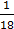# A thin convex lens L (refractive index=1.5) is placed on a plane mirror M.  When a pin is placed at A, such that OA=18 cm, its real inverted image is formed at A itself, as shown in the figure.  When a liquid of refractive indexis put between the lens and the mirror, the pin has to be moved to A’, such that OA’=27 cm, to gets its inverted real image at A’ itself.  The value ofwill be(a)(b)(c)(d)## Question ID - 51303 :- A thin convex lens L (refractive index=1.5) is placed on a plane mirror M.  When a pin is placed at A, such that OA=18 cm, its real inverted image is formed at A itself, as shown in the figure.  When a liquid of refractive indexis put between the lens and the mirror, the pin has to be moved to A’, such that OA’=27 cm, to gets its inverted real image at A’ itself.  The value ofwill be(a)(b)(c)(d)3537

fL=18cm=0.5⇒ R=18cm=(==⇒ 2=3(2−=6−3=Next Question :

Vandana  drove her car for 30 kms due North. Then she turned left and drove for 40 kms. She then turned left again and drove yet another 30 kms. Again she turned left and drove the car 50 kms. How far do you think she actually drove her car from the initial position ?

(A) 10 kms                            (B) 50 kms

(C) 30 kms                            (D) None of these